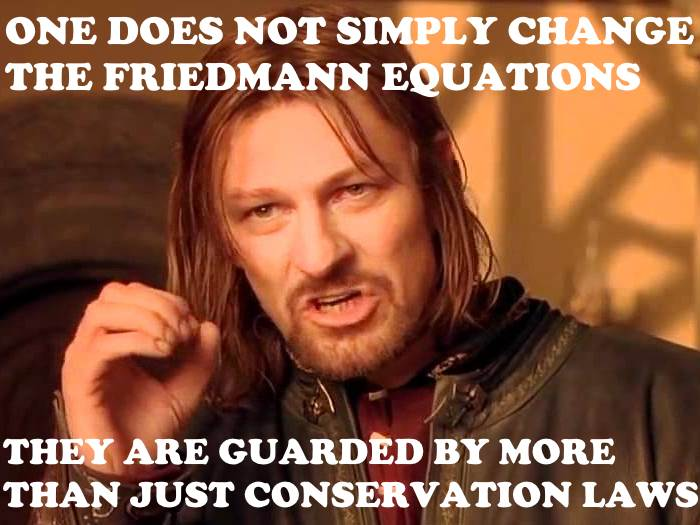Enough blogging about politics. It’s time to think about physics. Been a while since I last did that.

A Facebook post by Sabine Hossenfelder made me look at this recent paper by Josset et al. Indeed, the post inspired me to create a meme:The paper in question contemplates the possibility that “dark energy”, i.e., the mysterious factor that leads to the observed accelerating expansion of the cosmos, is in fact due to a violation of energy conservation.

Sounds kooky, right? Except that the violation that the authors consider is a very specific one.

Take Einstein’s field equation,

$$R_{\mu\nu}-\tfrac{1}{2}Rg_{\mu\nu}+\Lambda g_{\mu\nu}=8\pi GT_{\mu\nu},$$

and subtract from it a quarter of its trace times the metric. The trace of the left-hand side is $$-R+4\Lambda$$, the right-hand side is $$8\pi GT$$, so we get

$$R_{\mu\nu}-\tfrac{1}{4}Rg_{\mu\nu}=8\pi G(T_{\mu\nu}-\tfrac{1}{4}Tg_{\mu\nu}).$$

Same equation? Not quite. For starters, the cosmological constant $$\Lambda$$ is gone. Furthermore, this equation is manifestly trace-free: its trace is $$0=0$$. This theory, which was incidentally considered already almost a century ago by Einstein, is called trace-free or unimodular gravity. It is called unimodular gravity because it can be derived from the Einstein-Hilbert Lagrangian by imposing the constraint $$\sqrt{-g}=1$$, i.e., that the volume element is constant and not subject to variation.

Unimodular gravity has some interesting properties. Most notably, it no longer implies the conservation law $$\nabla_\mu T^{\mu\nu}=0$$.

On the other hand, $$\nabla_\mu(R^{\mu\nu}-\tfrac{1}{2}Rg^{\mu\nu})=0$$ still holds, thus the gradient of the new field equation yields

$$\nabla_\mu(\tfrac{1}{4}Rg^{\mu\nu})=8\pi G\nabla_\mu(T^{\mu\nu}-\tfrac{1}{4}Tg^{\mu\nu}).$$

So what happens if $$T_{\mu\nu}$$ is conserved? Then we get

$$\nabla_\mu(\tfrac{1}{4}Rg^{\mu\nu})=-8\pi G\nabla_\mu(\tfrac{1}{4}Tg^{\mu\nu}),$$

which implies the existence of the conserved quantity $$\hat{\Lambda}=\tfrac{1}{4}(R+8\pi GT)$$.

Using this quantity to eliminate $$T$$ from the unimodular field equation, we obtain

$$R_{\mu\nu}-\tfrac{1}{2}Rg_{\mu\nu}+\hat{\Lambda} g_{\mu\nu}=8\pi GT_{\mu\nu}.$$

This is Einstein’s original field equation, but now $$\hat{\Lambda}$$ is no longer a cosmological constant; it is now an integration constant that arises from a conservation law.

The vacuum solutions of unimodular gravity as the same as those of general relativity. But what about matter solutions? It appears that if we separately impose the conservation law $$\nabla_\mu T^{\mu\nu}$$, we pretty much get back general relativity. What we gain is a different origin, or explanation, of the cosmological constant.

On the other hand, if we do not impose the conservation law for matter, things get interesting. In this case, we end up with an effective cosmological term that’s no longer constant. And it is this term that is the subject of the paper by Josset et al.

That being said, a term that is time-varying in the case of a homogeneous and isotropic universe surely acquires a dependence on spatial coordinates in a nonhomogeneous environment. In particular, the nonconservation of $$T_{\mu\nu}$$ should lead to testable deviations in certain Parameterized Post-Newtonian (PPN) parameters. There are some reasonably stringent limits on these parameters (notably, the parameters $$\alpha_3$$ and $$\zeta_i$$ in the notation used by Clifford Will in the 1993 revision of his book, Theory and experiment in gravitational physics) and I wonder if Josset et al. might already be in violation of these limits.